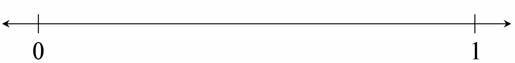Locating Fractions Less than One on the Number Line

Alignments to Content Standards: 3.NF.A.2

1. Mark and label the points $\frac14$, $\frac24$, $\frac34$, and $\frac44$ on the number line. Be as exact as possible.
2.3. Mark and label the point $\frac23$ on the number line. Be as exact as possible.
4.5. Mark and label the points $\frac12$, $\frac13$, $\frac14$, and $\frac15$ on the number line. Be as exact as possible.IM Commentary

In every part of this task, students must treat the interval from 0 to 1 as a whole, partition the whole into the appropriate number of equal sized parts, and then locate the fraction(s). The first part asks them to specifically identify the coordinate of all the partitions they create in the process of finding the unit fraction, where as the other parts just ask them to attend to finding one fraction for a given partition. Some students may find it helpful to use separate number lines to complete the last part of this task.

Students learn to use the number line to represent whole numbers in second grade, but this is the first time they will use the number line to represent fractions.

Some may have difficultly creating equal sized intervals, especially for thirds and fifths. Providing strategies, such as folding a separate piece of paper, might be helpful.

The following lists related tasks in order of sophistication:

• Locating Fractions Less than One on the Number Line
• Locating Fractions Greater than One on the Number Line
• Closest to $\frac12$
• Find 1
• Find $\frac23$
• Which is Closer to 1?

Solution

1.2.3.